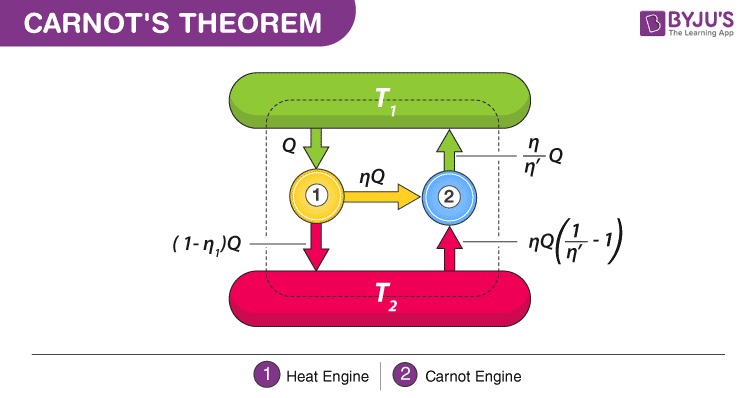# Carnot's Theorem

## What is Carnot’s theorem?

Carnot’s theorem also known as Carnot’s rule was developed by Nicolas Léonard Sadi Carnot in the year 1824, with the principle that there are limits on maximum efficiency for any given heat engine. It depends mainly on hot and cold reservoir temperatures.Carnot’s theorem states that:

• Heat engines that are working between two heat reservoirs are less efficient than the Carnot heat engine that is operating between the same reservoirs.
• Irrespective of the operation details, every Carnot engine is efficient between two heat reservoirs.
• Maximum efficiency is given as:
$$\eta _{max} = \eta _{Carnot} = 1-\frac{T_{c}}{T_{H}}$$

Where,

TC: absolute temperature of the cold reservoir

TH: absolute temperature of the hot reservoir

𝜼: the ratio of work done by the engine to heat drawn out of the hot reservoir

Processes involved in thermodynamics can be carried out in the following two ways:

Reversible engine:

The efficiency of all reversible engines remains the same that works between two same heat reservoirs.

$$\Delta S = \int_{a}^{b}\frac{dQ_{rev}}{T}$$

Where,

ΔS: change in entropy

T: temperature

$$\int_{a}^{b}$$ : to show that this is a path function

Irreversible engine:

There is no irreversible engine that is more efficient than the Carnot engine working between two same reservoirs.

Example of an irreversible engine are:

• Plastic deformation
• Friction
• Spontaneous chemical reaction

### Applications of Carnot’s theorem

• Carnot’s theorem finds application in engines that convert thermal energy to work.
• Refrigeration: Method of removal of heat from the at low temperature and dissipating it to a higher temperature. This is a reversible process.

Stay tuned with BYJU’S to learn more about the Carnot engine and other related topics with video lectures and interactive sessions.

Related Physics Articles:

## Carnot’s Theorem Important Questions

Q1. What working fluid is used in a Carnot cycle?

Ans: An ideal gas is used as a working fluid in a Carnot cycle.

Q2. What is the COP of a reversible heat pump?

Ans: We know that,

$$\frac{Q_{1}}{Q_{2}}=\frac{T_{1}}{T_{2}}$$

Therefore, COP=$$\frac{T_{1}}{T_{1}-T_{2}}$$

Q3. What is the COP for a reversible refrigerator?

Ans: We know that,

$$\frac{Q_{1}}{Q_{2}}=\frac{T_{1}}{T_{2}}$$

Therefore, COP=$$\frac{T_{2}}{T_{1}-T_{2}}$$

Q4. What is the efficiency of the reversible heat engine?

Ans: We know that,

Efficiency=$$1-\frac{Q_{2}}{Q{1}}$$

Therefore, the efficiency of the reversible heat engine is $$1-\frac{T_{2}}{T{1}}$$.

Q5. Give examples of a reversed heat engine.

Ans: Following are the examples of heat engine:

• Heat pump
• Refrigerator

Q6. What is diesel cycle?

Ans: Diesel cycle consists of one constant pressure, one constant volume and two isentropic processes.

Q7. What is the efficiency and work ratio of a simple gas turbine cycle?

Ans: The ratio between the efficiency and work ratio is very low.

Q8. Name the gas that has a minimum molecular mass.

Ans: Hydrogen has a minimum molecular mass.

Q9. Name the fuel used in steam boiler.

Ans: Non-coking bituminous coal is the fuel used in a steam boiler.

Q10. What is the relationship between gas constant and specific heat?

Ans: Gas constant is equal to the difference between two specific heats.

Test your knowledge on Carnot's theorem